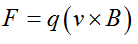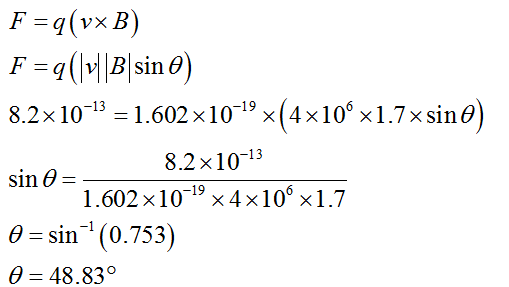# A proton moving at 4.00 × 106 m/s through a magnetic field of magnitude 1.70 T experiences a magnetic force of magnitude 8.20 × 10−13 N. What is the angle between the proton’s velocity and the field?

Question
13 views

A proton moving at 4.00 × 106 m/s through a magnetic field of magnitude 1.70 T experiences a magnetic force of magnitude 8.20 × 10−13 N. What is the angle between the proton’s velocity and the field?

check_circle

Step 1

Given:

Velocity of proton (v) = 4×106 m/s.

Magnitude of magnetic field (B) = 1.7 T.

Magnitude of magnetic force (F) = 8.2×10-13 N.

Charge on proton (q) = 1.602×10-19 C.

Step 2

The force exerted on moving proton in the magnetic field is given as,Step 3

Let, Angle between velocity and the magnetic field is θ,...

### Want to see the full answer?

See Solution

#### Want to see this answer and more?

Solutions are written by subject experts who are available 24/7. Questions are typically answered within 1 hour.*

See Solution
*Response times may vary by subject and question.
Tagged in